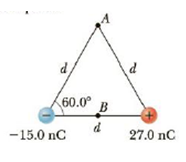Chapter 16, Problem 12P

Chapter
Section
Textbook Problem

The two charges in Figure P16.12 are separated by d = 2.00 cm. Find the electric potential at (a) point A and (b) point B, which is hallway between the charges.Figure P16.12

(a)

To determine
The potential at A.

Explanation

Formula to calculate the potential is,

VA=keq+d+keqd

• ke is the Coulomb constant.
• q+ and q are the charges.

Substitute 27.0 nC for q+ , 15.0nC for q , 2.00 cm for r and 8.99×109 Nm2C2 for ke .

VA=(8.99×109 Nm2C2)(27.0nC)2.00cm+(8.99×109 Nm2C2)(15

(b)

To determine
The potential at B.

Still sussing out bartleby?

Check out a sample textbook solution.

See a sample solution

The Solution to Your Study Problems

Bartleby provides explanations to thousands of textbook problems written by our experts, many with advanced degrees!

Get Started